# Projects Gallery

This document is always under construction.

## Contrast Sensitivity of Luminance Fields

The human eye does not perceive luminance fields as they really are. The contrast sensitivity function of the eye emphasizes intermediate spatial frequencies more than either lower or higher frequencies. One result is the appearance of "Mach bands" at all the sharp boundaries in a perceived field.

## Perturbation Bounds for Linear Regression Problems

This paper examines errors in the estimated solution vectorto the linear regression problem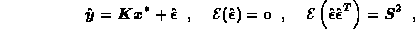when the dominant uncertainties are the measuring errors. Backward error analysis gives the hopelessly pessimistic bound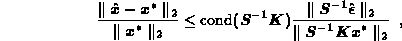by assuming the worst possible combination of random errors, an extremely unlikely occurence for nontrivial problems. The plots below show two estimates of the solution to a 150 x 121 linear regression problem obtained by discretizing a well known ill-posed problem and adding random errors obtained by sampling from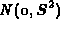with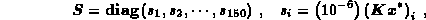which means that the errors in the elements of the right hand side vector were in the 6th digit. The dashed curve in each plot is the true solution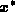, and the jagged solid curve is the estimated solution. The upper plot left was calculated in double precision with machine epsilon = 2.22E-16, and lower one was calculated in single precision with machine epsilon = 1.19E-7. Since the condition number of the matrix was 2.9E+9, the conventional interpretation of the above perturbation bound suggests that the double precision estimate should be accurate to 6 digits, but the single precision estimate should not contain any digits of accuracy. Yet the two are graphically identical!

###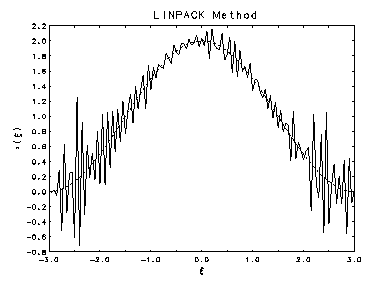Single Precision

A statistical perturbation analysis of the linear regression problem yields a much more realistic bound for the uncertainties in the estimated solution. The expected uncertainty in a single element of the estimate is shown to depend not on the condition number of the matrix, but rather on its smallest singular value. For the problem illustrated above, the Wilkinson perturbation approach gave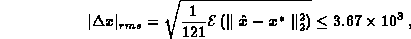while the statistical perturbation approach gave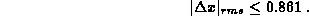The latter value is much more realistic that the former, but it exceeds the true value by a factor of 2.85. Actually, the whole perturbation approach is rather irrelevant since classical regression theory provides confidence intervals for the individual elements of the estimate which modern least squares algorithms could easily compute by inverting an upper triangular matrix formed in computing that estimate. Unfortunately the numerical analysis community seems to be unaware of this fact, and the least squares subroutines in the widely used LINPACK and LAPACK collections do not return confidence intervals, or even the variance matrix needed to compute them.

For more information, see: Rust, Bert W. (1994) "Perturbation Bounds for Linear Regression Problems", (postscript, 236K bytes), Computing Science and Statistics, 26, Interface Foundation of North America, pp. 528-532.

or: "interface94.ps.gz", (zipped postscript, 68.8K bytes).

## Linear Ill-Posed Problems

Fredholm integral equations of the first kind are used to model measurements obtained with an instrument which distorts and/or smooths the function being measured. The kernel models the instrument response function and the right hand side function models the measurements obtained. Unfortunately, real instruments also add random measurement errors to the observed right hand side. These uncertainties, no matter how small, make a stable inversion of the integral equation impossible. Small errors in the observations produce huge errors in the solution of the integral equation -- hence the name "ill-posed problems." When such a problem is discretized, in order to compute a numerical solution, the result is an ill-conditioned linear regression problem which can be made to yield physically reasonable estimates only by imposing physically motivated side constraints on the problem.

#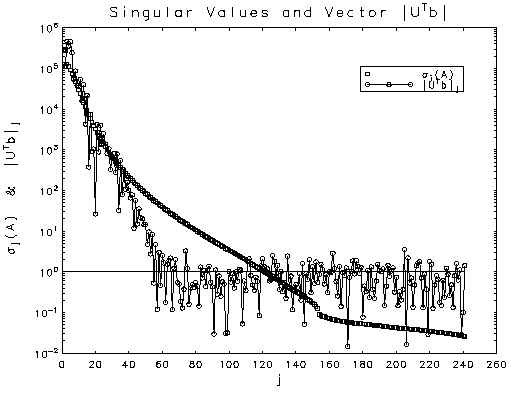Discretizing an ill-posed problem yields an ill-conditioned linear regression model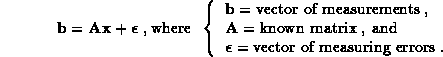Least squares gives a wildly unstable estimate ofand a sum of squared residuals much smaller than the expected value. Apparently the estimate captures part of the variance that properly belongs in the residuals. One strategy for stabilizing the estimate by shifting some of this variance to the residuals is to truncate the singular value decomposition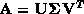where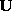and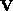are orthogonal and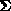is a diagonal matrix of singular values. Singular values below some threshold are reset to zero to give a new diagonal matrix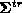, and the estimate is calculated from the generalized inverse of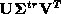. The most delicate part of this procedure is the determination of the truncation threshold. Conventionally this has been regarded as a problem of determining the ``numerical rank'' of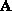, but in most casesis clearly not rank-deficient. This paper suggests an alternate strategy which uses the variances of the measurement errors to specify a truncation for the elements of the vector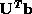. The idea is to zero the elements dominated by measurement errors and compute the estimate using the full rank matrix. The problem of setting the truncation threshold becomes equivalent to deciding which of the rotated measurments are significantly different from zero. Plotted above against element number are the singular values (squares) and the absolute values of the elements of(connected circles) for a problem which has been scaled so that the elements ofare idependently and identically distributed with the standard normal distribution. These values were computed with an arithmetic precision of 2.22E-16 so the matrix is clearly not rank deficient. The dichotomy in the distribution of the elements ofindicates the level of measuring error saturation, with the horizontal line marking the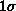level. Choosing a truncation level of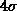gives a stable estimate and an acceptable value for the sum of squared residuals.

For more information, see: Rust, Bert W., Truncating the Singular Value Decomposition for Ill-Posed Problens , (postscript, 2.57M bytes), NISTIR 6131, U.S. Dept. of Commerce, July 1998.

or: MS-TruncSVD.ps.gz, (zipped postscript, 555K bytes).

## Confidence Intervals for Discrete Approximations to Ill-Posed Problems

The linear, first-kind integral equations which model many physical measurement processes constitute an ill-posed problem when the measurement errors are taken into account. When discretized, they produce ill-conditioned linear regression models whose solutions are pathologically sensitivite to even very small errors. Stable solutions can often be found by imposing physically motivated nonnegativity constraints. Pictured below are plots of estimated one-at-a-time, 95% confidence intervals for the flux of monoenergetic neutrons from the nuclear reaction T (d,n) 4He. Although the expected peak at 14 MeV is discernible in the top plot, which shows the classical unconstrained confidence intervals, it is accompanied by three other spurious peaks, and most of the lower bounds and some of the uppper bounds are negative. The lower plot shows the bounds obtained when a nonnegativity constraint is imposed on the solution. The result is a very clean energy spectrum dominated by the expected peak at 14 MeV.

###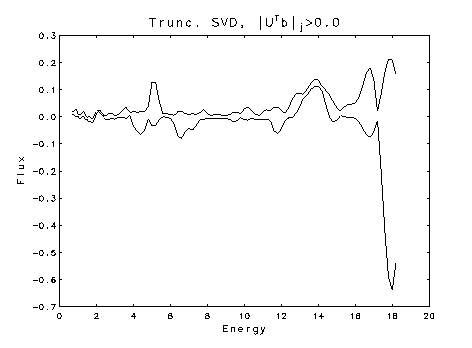Flux unconstrained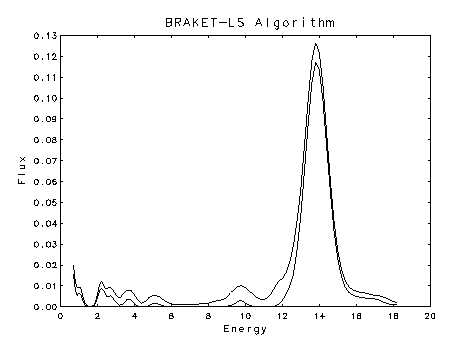Flux nonnegative

The method for computing nonnegatively constrained, simultaneous confidence intervals has been known for some time (Burrus, W.R., Utilization of a priori information by means of mathematical programming in the statistical interpretation of measured distributions, ORNL-3743, June 1965). The extension of the method to compute one-at-a-time invtervals is described in: Rust, B.W. and O'Leary, D.P., "Confidence intervals for discrete approximations to ill-posed problems," Jour. Comp. Graph. Statistics, 3 (1994) pp. 67-96. The abstract for this paper is given below:

We consider the linear model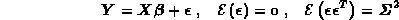obtained by discretizing a system of first-kind integral equations describing a set of physical measurements. The n-vector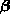represents the desired quantities, the m x n matrix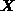represents the instrument response functions, and the m-vector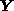contains the measurements actually obtained. These measurements are corrupted by random measuring errorsdrawn from a distribution with zero mean vector and known variance matrix. Solution of first-kind integral equations is an ill-posed problem, so the least squares solution for the above model is a highly unstable function of the measurements, and the classical confidence intervals for the solution are too wide to be useful. The solution can often be stabilized by imposing physically motivated nonnegativity constraints. In a previous paper (O'Leary, D.P. and Rust, B.W., SIAM J. Sci. Stat. Comput., 7, 1986, pp. 473-489) we developed a method for computing sets of nonnegatively constrained simultaneous confidence intervals. In this paper we briefly review the simultaneous intervals and then show how to compute nonnegativity constrained one-at-a-time confidence intervals. The technique gives valid confidence intervals even for problems with m < n. We demonstrate the methods using both an overdetermined and an underdetermined problem obtained by discretizing an equation of Phillips (Phillips, D.L., J. Assoc. Comput. Mach., 9, 1962, pp. 84-97).

An extended version of this paper, which gives more details and includes a real world example, can be found in: Rust, B.W. and O'Leary, D.P., Confidence Intervals for Discrete Approximations to Ill-Posed Problems, NISTIR 4720, U.S. Dept. of Commerce, Jan. 1992.

## Global Temperatures, Gaia, and Fossil Fuel Production

It is now generally accepted that the Earth is warming because of an enhancement of the greenhouse effect caused by increasing emissions of carbon dioxide from fossil fuel consumption. The circles in the plot below represent the global, yearly fossil fuel production totals for the indicated years. The dashed line is a nonlinear least squares fit of an exponential function to the data. The departures of the measured values from this exponential baseline are inversely proportional to global temperature variations. The solid line is the fit of a model which which takes this temperature modulation into account. This inverse modulation represents a feedback consistent with the Gaia hypothesis for planetary greenhouse temperature control. The model predicts that increasing temperatures will tend to suppress the growth of fossil fuel production.

#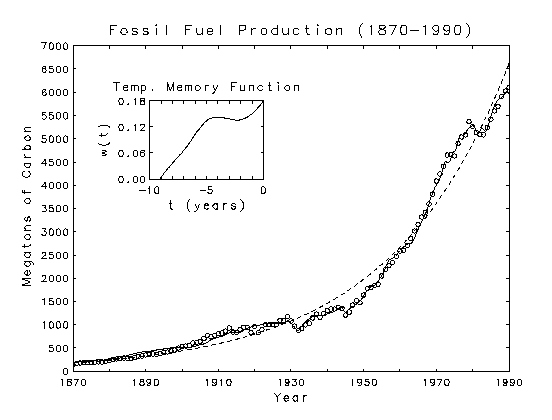More details about this temperature modulation model are given in the paper: Rust, B.W. and Crosby, F.J., "Further studies on the modulation of fossil fuel production by global temperature variations," Environment International, 20 (1994) pp. 429-456. The abstract of this paper is given below:

We extend the earlier work of Rust and Kirk (Rust, B.W. and Kirk, B.L., Environment International, 7, 1982, pp. 419-422) on the inverse modulation of global fossil fuel production by variations in Northern Hemispheric temperatures. The present study incorporates recent revisions and extensions of the fuel production record and uses a much improved temperature record. We show that the new data are consistent with the predictions of the original Rust-Kirk model which we then extend to allow for time lags between variations in the temperature and the corresponding responses in fuel production. The modulation enters the new model through the convolution of a lagged averaging function with the temperature time-series. We also include explicit terms to account for the perturbations caused by the Great Depression and World War II. The final model accounts for 99.84% of the total variance in the production record. The temperature modulation produces variations of as much as 30% in the total production. This modulation represents a feedback which is consistent with the predictions of the Gaia hypothesis for a planetary greenhouse temperature control. We use the new model to calculate 20-year fuel production predictions for three temperature scenarios which hopefully bracket the possibilities for temperature behavior during that time.

## Separating Signal from Noise in Global Warming

### Reprinted from Computing Science and Statistics, Volume 35 (2003) pp. 263-277.

Abstract: One argument often used against global warming is that the global temperature record is too noisy to allow a clear determination of the signal. This paper presents two models for the signal which suggest that: (1) the warming is accelerating, (2) the warming is related to the growth in fossil fuel emissions, and (3) the warming in the last 146 years has been at least 10 times greater than the noise level. One model uses a constant rate for the acceleration and the other an exponential whose rate constant is exactly one half that of the growth in fossil fuel emissions. The two models can be viewed as best case and worst case scenarios for extrapolations into the future, but the data measured so far cannot reliably distinguish between them.

## Carbon Dioxide, Global Warming, and Michael Crichton's "State of Fear"

### To be published in Computing Science and Statistics , Vol. 37.

Abstract: In his recent novel, State of Fear [HarperCollins, 2004], Michael Crichton questioned the connection between global warming and increasing atmospheric carbon dioxide by pointing out that for 1940-1970, temperatures were decreasing while atmospheric carbon dioxide was increasing. A reason for this contradiction was given at Interface 2003 [ B.W. Rust, Computing Science and Statistics, Vol. 35 (2003) pp. 263-277 ] where the temperature time series was well modelled by a 64.9 year cycle superposed on an accelerating baseline. For 1940-1970, the cycle decreased more rapidly than the baseline increased. My analysis suggests that we are soon to enter another cyclic decline, but the temperature hiatus this time will be less dramatic because the baseline has accelerated. This paper demonstrates the connections between fossil fuel emissions, atmospheric carbon dioxide concentrations, and global temperatures by presenting coupled mathematical models for their measured time series.

####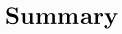The plots just below have been reconstructed to look like the ones at the top of page 86 in Michael Crichton's novel State of Fear. It was necessary to manually digitize the data curves in the book because he did not cite a source for the "CO2 Levels" curve, and the sources cited for the "Annual Mean" curve of temperature anomalies do not correspond to the data actually plotted. Also, the "5-year Mean" curve appears to be a plot of 11-year running means. He included these plots in order to allow one of his characters, on page 87, to pose the question: So, if rising carbon dioxide is the cause of rising temperatures, why didn't it cause temperatures to rise from 1940 to 1970? The book also contains many other time series plots of local temperature anomalies in various cities around the world, many of them showing definite cooling rather than warming trends. He uses all these plots to argue that global warming is a hoax foisted on the world by environmentalists with a secret agenda. This message has been warmly received by some high government officials and conservative pundits, but has been sharply criticized by others more familiar with the measurements.

####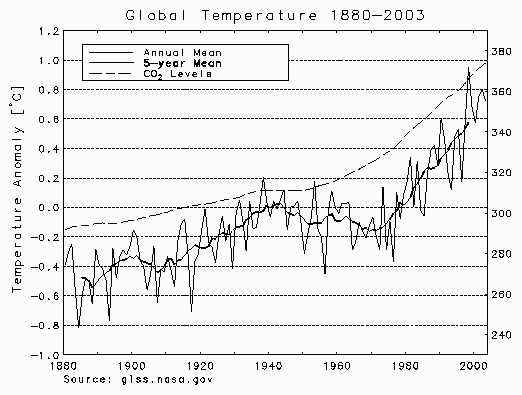Crichton's plot on page 86

The data which presumably were the source for the "CO2 Levels" curve are plotted as discrete points in the plot below. The small solid circles are annual average atmospheric concentrations of CO2 measured, in parts per million by volume [ppmv], by C. D. Keeling and his colleagues at Mauna Loa in Hawaii. These averages, which were computed from very precise daily measurements, are so accurate that their uncertainty intervals are smaller than the size of the dots used to plot them. The open squares and diamonds are proxy measurements obtained from air bubbles trapped in the ice at two sites in Antarctica. They are not nearly so accurate as Keeling's measurements, and there are uncertainties of ± 2 years in their measured dates because the air bubbles can migrate in the ice and snow from which it is compacted. Crichton's curve, which is plotted with a dashed line pattern, follows the Keeling data closely and the proxy data fairly well.

###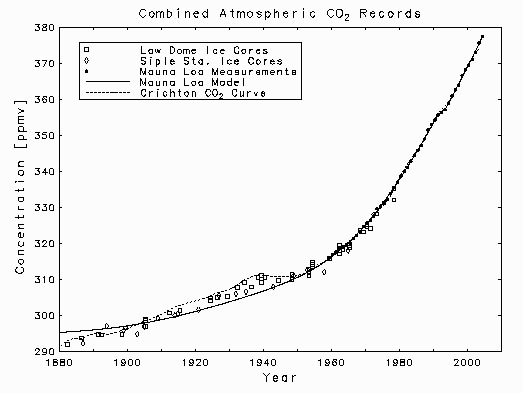Atmospheric carbon dioxide concentrations

The solid curve in the above plot is the linear least squares fit to the Mauna Loa measurements of the model

####The function P(t) is the rate of fossil fuel emissions of CO2 to the atmosphere, which is plotted in the graph below, and S(t) is a linear, two year, unit ramp function used to model the eruption of Mt. Pinatubo in June 1991, i.e.,

####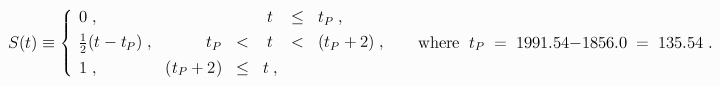The emissions data used to define the function P(t) are plotted, in units of megatons of carbon per year, as discrete points below, where the solid curve through them is the least squares fit of the model

####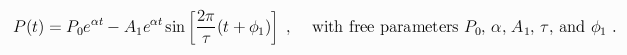This function, which is just a superposition of an exponential baseline and a sinusoid whose amplitude grows with the same exponential rate, was used to calculate the integral in the above model for c(t). The estimates for the P(t) parameters were

####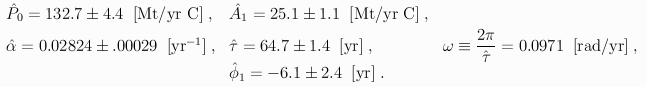The fit accounts for 99.76% of the variance in the data in spite of the fact that it could not accommodate the striking perturbations due to the Great Depression, the two World Wars and the OPEC oil supply disruptions.

When the above parameter estimates were used to define the function P(t) in the above expression for c(t), the fit of that function to Keeling's atmospheric CO2 measurements gave the parameter estimates

####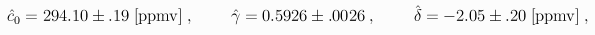and the fit explained 99.97% of the variance in the data. The plot above shows that when the fit is extrapolated back to 1880.0, it tracks the ice core proxy data quite well, even though the latter were not used in obtaining it. Therefore it is surely a more accurate representation of the real atmospheric concentrations than Cricthton's unexplained "CO2 Levels" curve. The parameter estimates show that &asymp 60% of the CO2 emitted to the atmosphere remains there and that Mt. Pinatubo caused a decrease, clearly visible in the data, of &asymp 2 [ppmv] in the atmospheric concentration. This decrease has been shown by Gu, et al. [Science, Vol. 299 (2003) pp. 2035-2038] to have been caused by enhanced terrestrial photosynthesis due to an aerosol-induced increase in diffuse solar radiation. The c(t) fit and back extrapolation has been replotted in the figure below for comparison with the P(t) fit. Note that the integral in the c(t) model smooths out the 65-year P(t) cycle, but the c(t) fit still tracks the exponential P(t) baseline quite well.

###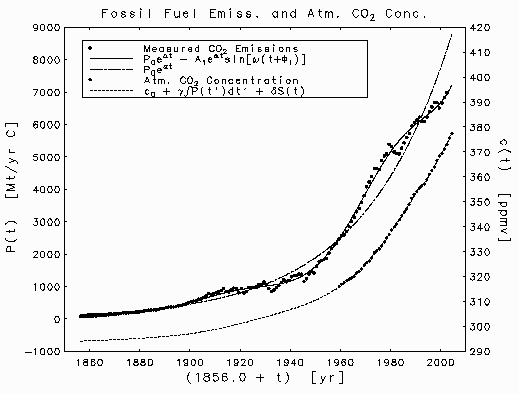Fossil fuel emissions of carbon dioxide

Crichton's annual global average temperature anomaly curve is replotted in the figure below. In the text he refers the reader to an Appendix where he claims that

World temperature data has been taken from the Goddard Institute for Space Studies, Columbia University, New York (GISS); the Jones, et al. data set from the Climate Research Unit, University of East Anglia, Norwich, UK (CRU); and the Global Historical Climatology Network (GHCN) maintained by the National Climatic Data Center (NCDC) and the Carbon Dioxide Information and Analysis Center (CDIAC) of Oak Ridge National Laboratory, Oak Ridge, Tennessee.
In fact, his curve does not correspond to any of the annual global average data sets given by these sources. The GISS and CRU records have been plotted as discrete data points in the figure below. In each case, the reference temperature for the anomalies has been readjusted to minimize the mean square difference between the record and Crichton's curve. These zero-point adjustments brought the GISS and CRU anomalies into good agreement with one another, but neither record is concordant with Crichton's curve. There is good agreemnt in the middle of the records, particularly in the years 1940-1970, but at the beginning and end, Crichton's curve displays significant departures from all of the records which he cited as sources. In particular, his curve completely misses another period of cooling in the years 1880-1910. Had he noticed that, he might have discovered the answer to his question about the cooling in the years 1940-1970.

###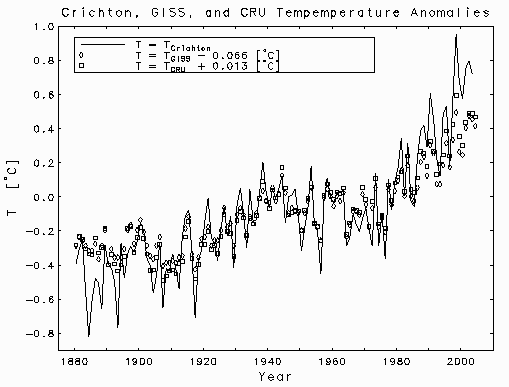Crichton, GISS, and CRU anomalies

The answer to Crichton's question was actually given ten years before he posed it by Schlesinger and Ramankutty [Nature, Vol. 367 (1994) pp. 723-726] who reported An oscillation in the global climate system of period 65-70 years. The CRU estimates of annual global average temperature anomalies are plotted below, as discrete open circles, together with the linear least squares fits of the three mathematical models:

####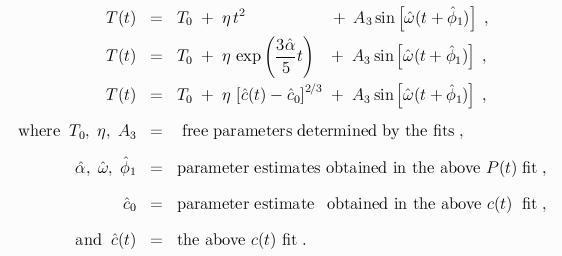Each of the three models consists of a sinusoid, with period 64.7 years, oscillating around an accelerating baseline. It is remarkable that such good fits to the T(t) time series were obtained using a sinusoid with exactly the same period and phase that were determined for the oscillation in the P(t) data! This does not mean that the temperature oscillation is caused by the oscillation in the emissions. The sinusoids appear with their signs reversed in the two models, so higher emissions correspond to lower temperatures and lower emissions to higher temperatures. This inverse relation between the variations in the two quantities was first noticed in 1978 by Rust and Kirk [Environment International, Vol. 7 (1982) pp.419-422] who modelled it with the differential equation

####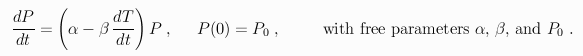They did not notice that the variations in the two records were sinusoidal oscillations, so they used a smoothing spline approximation to the rather crude temperature record that was available at the time, but still got a good fit to the emissions data.

###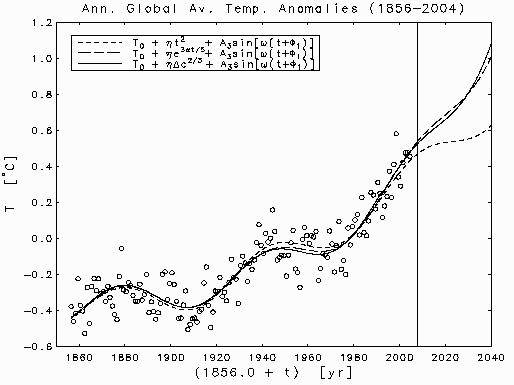CRU's temperature anomalies

The three accelerating baselines used in the above temperature models do not exhaust all the possibilities, but they all represent behavior that is common in nature. The quadratic baseline represents constant acceleration. Adding a linear term to that model does not produce a statistically significant reduction in the sum of square residuals, so the data seem to demand a monotonically increasing baseline.

The rate constant for the exponential baseline, &alpha &prime = 3 &alpha / 5, was chosen, somewhat arbitrarily, from the interval [0.0146 &le &alpha &prime &le 0.0190] yr -1. Changing it to any other value in that interval would work just as well because the estimate for &eta would also change in just the right way to produce almost the same fitted curve.

The most interesting of the three models is the one with the baseline given by a power law relation between changes in temperature and changes in atmospheric CO2 concentration. The power &nu = 2/3 was chosen, again somewhat arbitrarily, from the interval [0.582 &le &nu &le 0.708]. Any other value in the interval would have worked about as well, with the estimate of &eta changing in just the right way to give almost the same fitted curve.

Each of the three models explained &asymp 85% of the variance in the data, so there is no good way to choose between them. Although they give similar fits to the currently available data, they extrapolate into the future quite differently. The next maximum of the temperature cycle will occur in September 2007 and the next minimum in March 2040. Assuming that the present rate of increase in fossil fuel production can be maintained implies that the approaching cooling period will not be so pronounced as the ones that started in &asymp 1880 and in &asymp 1940. Only the constant acceleration, quadratic baseline model would produce a noticeable hiatus in the rising temperatures.

## Fitting Nature's Basic Functions

This is a series of articles, published in Computing in Science and Engineering, on the art of least squares modelling of measured time series data using combinations of the most basic functions governing the dynamics of natural processes, with a particular emphasis on cyclical and exponential behavior. The modelling techniques are applied to the historical records of global total fossil fuel carbon dioxide emissions, atmospheric carbon dioxide abundance, and global average temperatures of the lower troposphere. Standard statistical diagnostics are used to assess the models and the connections between them. Short-term extrapolations into the future are considered.

### Fitting Nature's Basic Functions Part I: Polynomials and Linear Least Squares

This installment explains linear least squares with a special emphasis on polynomial fits. It describes the statistical assumptions in the general linear model and explains why the least squares calculation gives the best linear unbiased estimate for the unknown parameters. It uses the meaured global average annual temperature record for the years 1856-1999 as an example data set and shows that a polynomial of degree at least five is required to capture the systmatic variation in the record.

####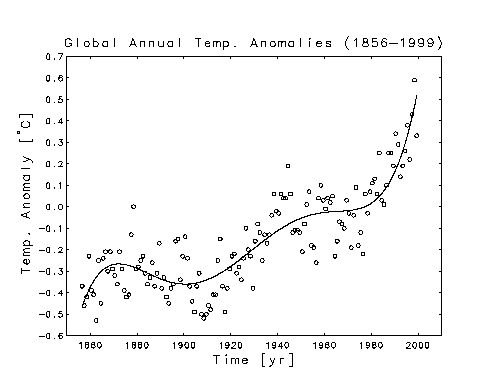The Temperature Data

Unfortunately, the final published manuscript had some errors. Corrections can be obtained from Errata for Part I

### Fitting Nature's Basic Functions Part II: Estimating Uncertainties and Testing Hypotheses

This installment explains statistical diagnostic techniques for evaluating linear least squares fits. Topics covered include the variance and correlation matrices for the least squares estimate, construction of confidence intervals for the estimate, and testing hypotheses about the statistical significance of the individual components of the estimate. The use of the techniques is illustrated by applying them to the analysis of polynomial fits to the meaured global average annual temperature record for the years 1856-1999. In particular it is shown that for a quadratic polynomial fit, the coefficient of the linear term is not statistically significant. The solid curve in the plot below represents a full quadratic fit, with three adjustable paramenters, and the dashed curve represents a reduced quadratic fit, with the parameter for the linear term omitted. Including the linear parameter does not produce a statistically significant reduction in the is sum of squared residuals. This suggests that the baseline trend for the global warming is increasing monotonically.

### Fitting Nature's Basic Functions Part III: Exponentials, Sinusoids, and Nonlinear Least Squares

This installment introduces nonlinear least squares fitting and describes the Gauss-Newton iteration and the Levenberg-Marquardt variant of it. It explains how to fit exponential and sinusoidal functions to measured data and how to compute statistical diagnostics and test hypotheses about the fits. It illustrates the techniques by fitting various nonlinear models to the the time series records of annual global total fossil fuel emissions for the years 1751-1998 and global annual average temperatures for 1856-2001.

####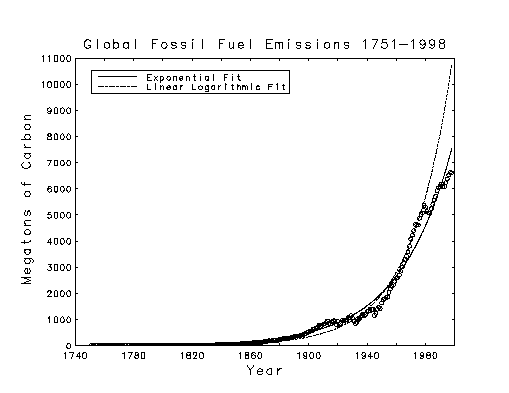Fossil Fuel Emissions Data

The linear logarithmic curve above was obtained from a linear least squares fit of a straight line to the logarithms of the data, and the exponential curve was obtained by nonlinear least squares fitting to the data itself. Both fits used 2 adjustable parameters.

All of the curves in the plot below were obtained by nonlinear least squares fitting of models with 5 adjustable parameters. In each case, three of the parameters defined a sinusoid with a period of approximately 65 years. The other two parameters defined a baseline. The reduced quadratic baseline had the same form as the one discussed in Part II. The fixed rate exponential baseline had the form of a constant plus an exponential whose rate was fixed at the value obtained from the above exponential fit to the fossil fuel emissions data. All of the terms in the 3 fits were statistically significant. The straight line baseline fit accounted for 75.8% of the total variance in the data, the exponential baseline fit accounted for 79.0%, and the reduced quadratic baseline fit accounted for 81.0%.